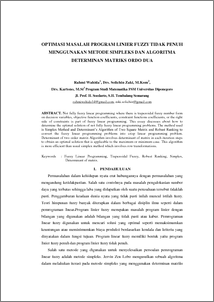# OPTIMASI MASALAH PROGRAM LINIER FUZZY TIDAK PENUH MENGGUNAKAN METODE SIMPLEKS DAN ALGORITMA DETERMINAN MATRIKS ORDO DUA

wahida, rahmi (2017) OPTIMASI MASALAH PROGRAM LINIER FUZZY TIDAK PENUH MENGGUNAKAN METODE SIMPLEKS DAN ALGORITMA DETERMINAN MATRIKS ORDO DUA. Undergraduate thesis, FAKULTAS SAINS DAN MATEMATIKA.Preview
PDF
427Kb

## Abstract

. Not fully fuzzy linear programming where there is trapezoidal fuzzy number form on decision variables, objective function coefficients, constraint functions coefficients, or the right side of constraints is part of fuzzy linear programming. This essay discusses about how to determine the optimal solution of not fully fuzzy linear programming problems. The method used is Simplex Method and Determinant’s Algorithm of Two Square Matrix and Robust Ranking to convert the fuzzy linear programming problems into crisp linear programming problem. Determinant of two order matrix Algorithm involves determinant of matrix in each iteration steps to obtain an optimal solution that is applicable to the maximum or minimum case. This algorithm is more efficient than usual simplex method which involves row transformations

Item Type: Thesis (Undergraduate) Q Science > Q Science (General) 50945 INVALID USER 09 Apr 2018 10:04 09 Apr 2018 10:04

Repository Staff Only: item control page